## 复现广发证券《基于日内高频数据的短周期选股因子研究》

1798人浏览 / 0人评论 2019-12-17

# 2 因子构建

1. 对于每个个股在交易日t，首先计算个股在特定分钟频率下第i个的收益率 rt,irt,i， rt,irt,i = pt,ipt,i − pt,i1pt,i−1，其中pt,ipt,i表示在交易日t，个股在第i个特定分钟频率下的对数价格，pt,i1pt,i−1表示在交易日t，个股在第i−1个特定分钟频率下的对数价格。

2. 对于每个个股，根据𝑟𝑡,𝑖分别计算个股在交易日t下的已实现方差(Realized Variance) RDVartRDVart、已实现偏度(Realized Skewness) RDSkewtRDSkewt，已实现峰度(Realized kurtosis) RDKurttRDKurtt。其中: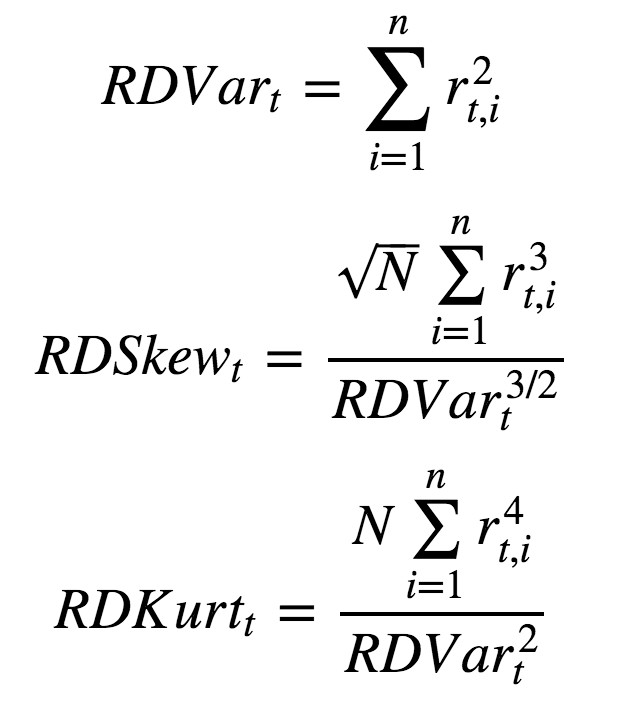1. 对于每个个股在交易日t计算累计已实现波动(Realized Volatility)RVoltRVolt， 已实现偏度(Realized Skewness)RSkewtRSkewt、已实现峰度(Realized Kurtosis) RKurttRKurtt ，其中: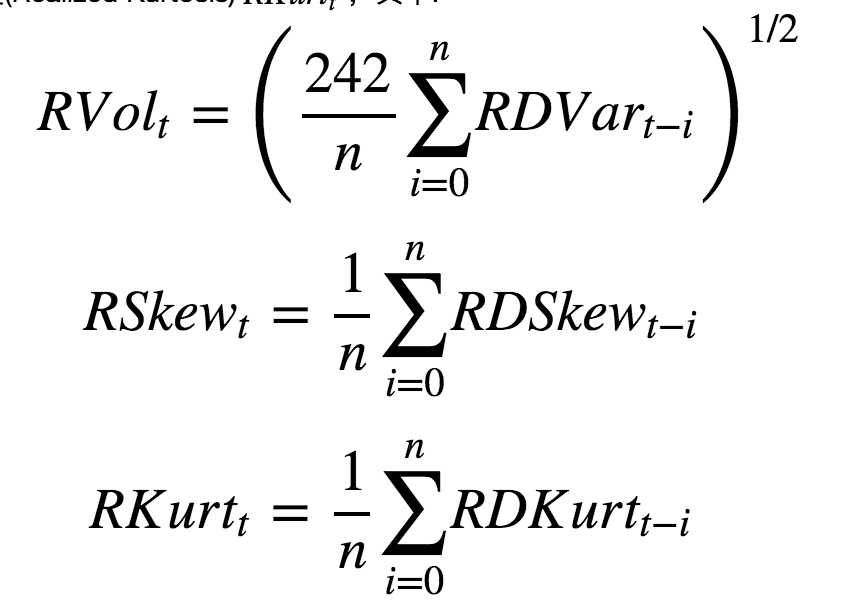1. 在每期调仓日截面上，按照上述公式计算每个个股的已实现波动(Realized Volatility)RVoltRVolt，已实现偏度(Realized Skewness)RSkewtRSkewt、已实现峰度(Realized Kurtosis)RKurttRKurtt指标，针对每个由高频数据计算得到的因子指标在历史上的分档组合表现，试图寻找出相对有效的因子指标。

# 3 构造因子数据

```import pandas as pd
import numpy as np
from jqdata import *
import math
from pandas import *展开代码 ↓    ```
```# 获取某个交易日的因子值
def get_one_trade_day_data(cache = {}, stocks = None, trade_date = None):

# 后面需要在交易日t计算累计已实现波动等数据，需要获取交易日t到交易日t-n之间的交易日展开代码 ↓    ```
```# 按照研报要求，去除上市不满一年的股票，剔除ST股
def filter_stock(stocks, date):
# 去除上市距beginDate不足6个月的股票
stock_list = []
for stock in stocks:展开代码 ↓    ```
```# 获取样本区间内的因子数据

# 研报中样本区间:2007年1月1日至2019年3月27日
# 2007-2019数据实在太多了，这里减一半，使用2013-2019，也一样涵盖牛市和熊市
start_date = date(2013, 1, 1)展开代码 ↓    ```
```2013-01-04
2013-01-11
2013-01-18
2013-01-25
2013-02-01展开输出 ↓    ```
```# 把计算出的因子数据写入文件 方便后续调用
import pickle

pkl_file = open('factor_dict.pkl', 'wb')
pickle.dump(factor_dict, pkl_file, 0)展开代码 ↓    ```

# 4 因子特征展示

## 1 频率分布

```import pickle

import matplotlib.pyplot as plt
import numpy as np

```market_vol = []
market_skew = []
market_kurt = []

for date in factor_dict:展开代码 ↓    ```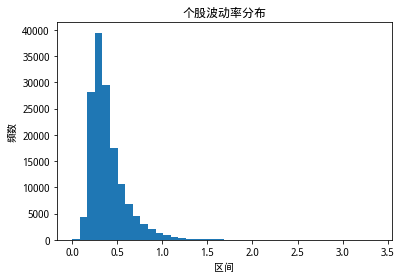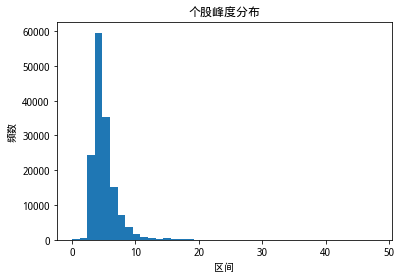## 2 百分位走势

```# 显示因子百分位走势
def show_percent_factor_value(factor_dict, factor_name, legend_loc):
# 百分位走势5档颜色 蓝 橙 绿 红 紫
color_list = ['#5698c6', '#ff9e4a', '#60b760', '#e05c5d', '#ae8ccd']

```# 'r_vol', 'r_skew', 'r_kurt'
show_percent_factor_value(factor_dict, 'r_vol', 'upper left')
show_percent_factor_value(factor_dict, 'r_skew', 'lower left')
show_percent_factor_value(factor_dict, 'r_kurt', 'upper left')
```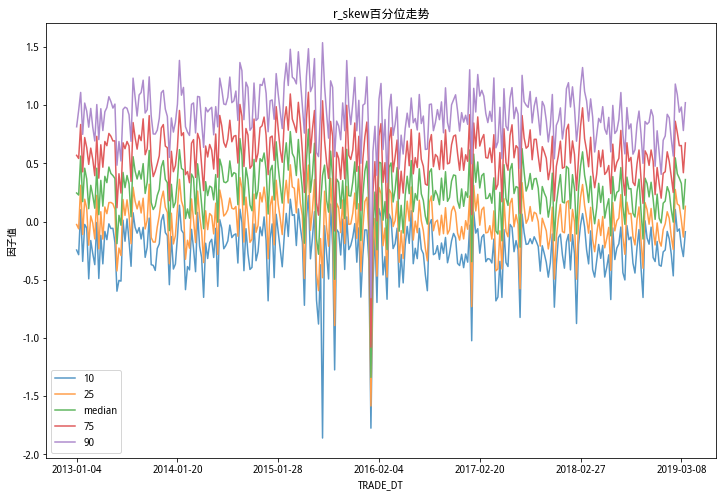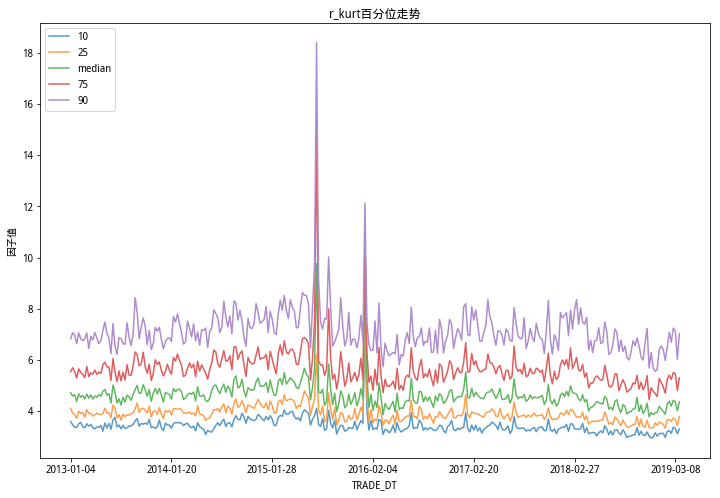# 5 实证分析

## 1 因子选股分档表现

```def group_df(df, count):
length = int(len(df) / count)

groups = []
for i in range(0, count - 1):展开代码 ↓    ```
```# 求每天的收益率
import pandas as pd

ret_df_index = []
ret_df_data = []展开代码 ↓    ```
```# 'r_vol', 'r_skew', 'r_kurt'
show_factor_level_return_rate(ret_df, factor_dict, 'r_vol')
show_factor_level_return_rate(ret_df, factor_dict, 'r_skew')
show_factor_level_return_rate(ret_df, factor_dict, 'r_kurt')
```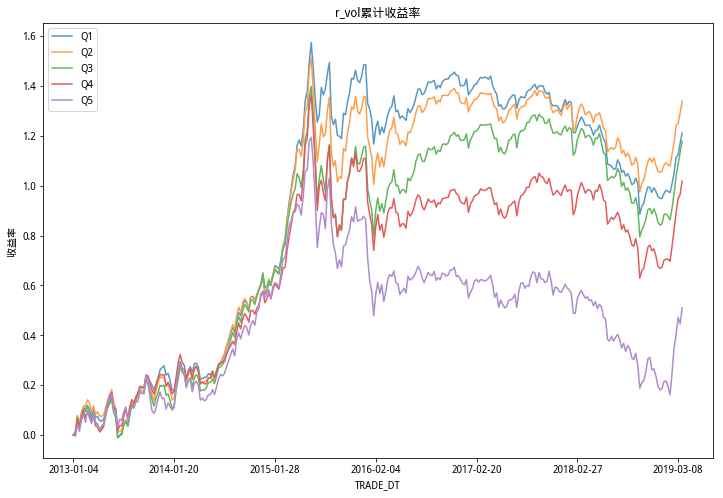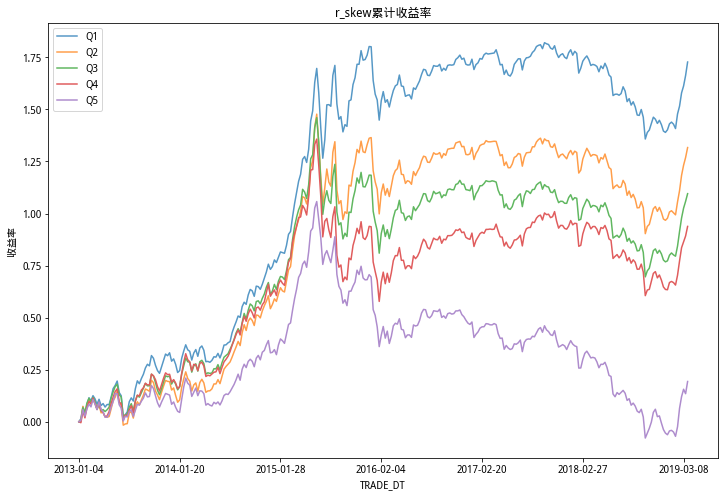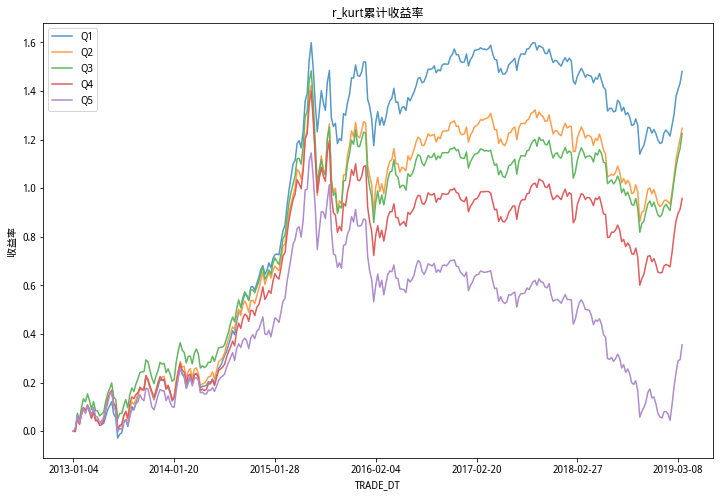## 2 因子多空收益

```# 展示某因子的多空收益率（Bull and Bear）
def show_factor_bb_return_rate(ret_df, factor_dict, factor_name):
color = 'red'
label = '多空累计收益'

```# 'r_vol', 'r_skew', 'r_kurt'
show_factor_bb_return_rate(ret_df, factor_dict, 'r_vol')
show_factor_bb_return_rate(ret_df, factor_dict, 'r_skew')
show_factor_bb_return_rate(ret_df, factor_dict, 'r_kurt')
```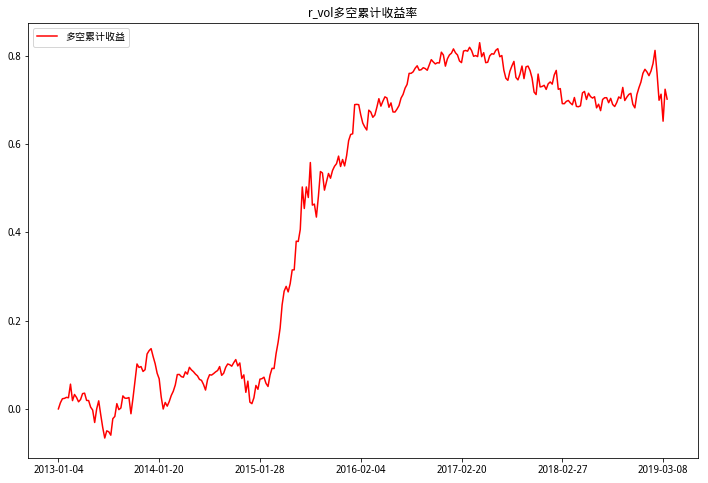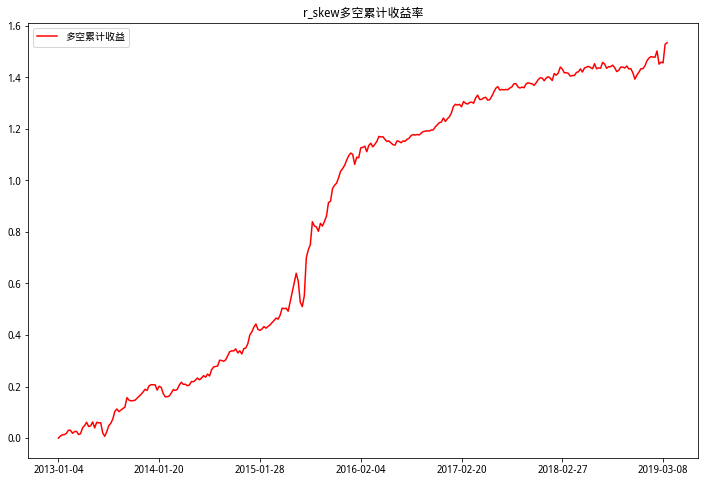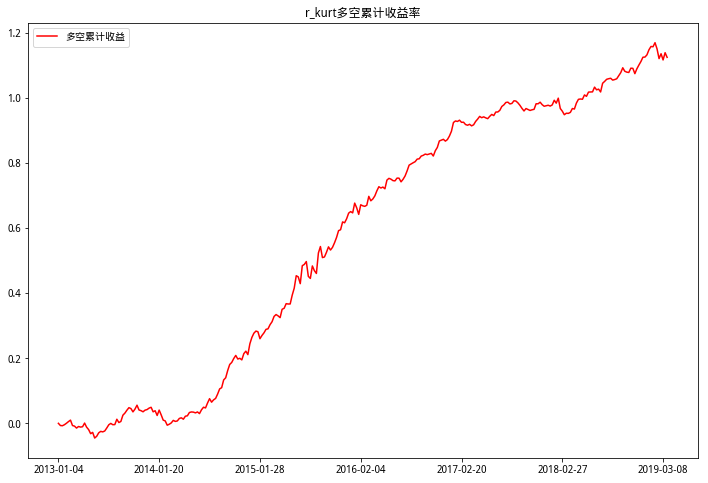## 3 因子IC

```import scipy.stats as st
# 展示某因子的IC
def show_factor_ic(ret_df, factor_dict, factor_name):
color_list = ['#2B4C80', '#B00004']

```# 'r_vol', 'r_skew', 'r_kurt'
show_factor_ic(ret_df, factor_dict, 'r_vol')
show_factor_ic(ret_df, factor_dict, 'r_skew')
show_factor_ic(ret_df, factor_dict, 'r_kurt')
```

```r_vol ic 小于0的个数占比：0.5973597359735974
```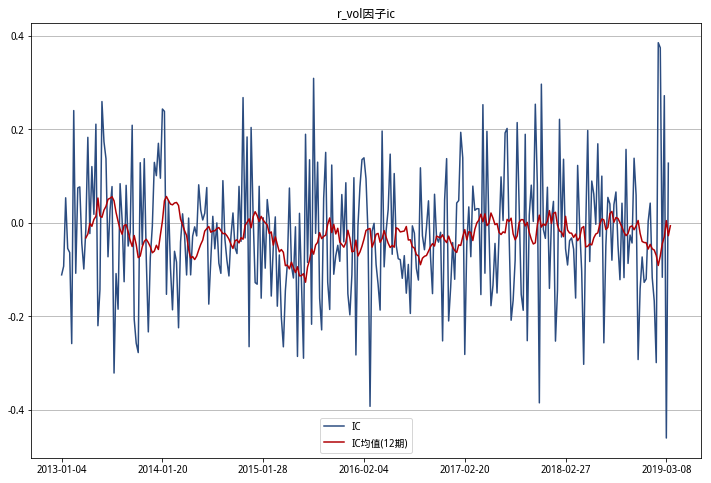```r_skew ic 小于0的个数占比：0.6600660066006601
```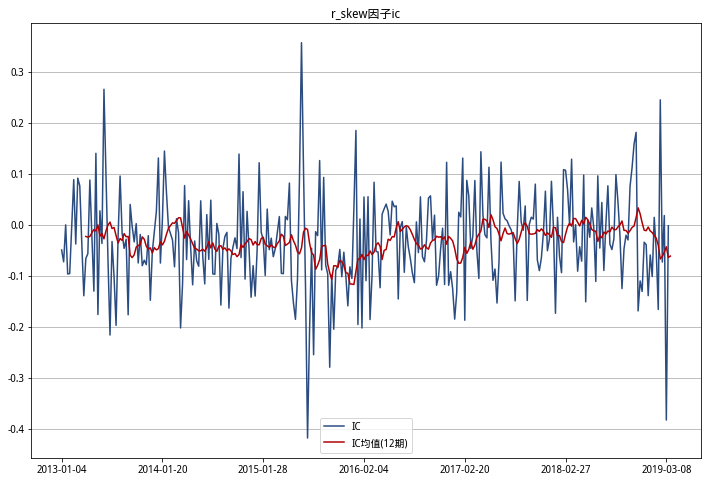```r_kurt ic 小于0的个数占比：0.66996699669967
```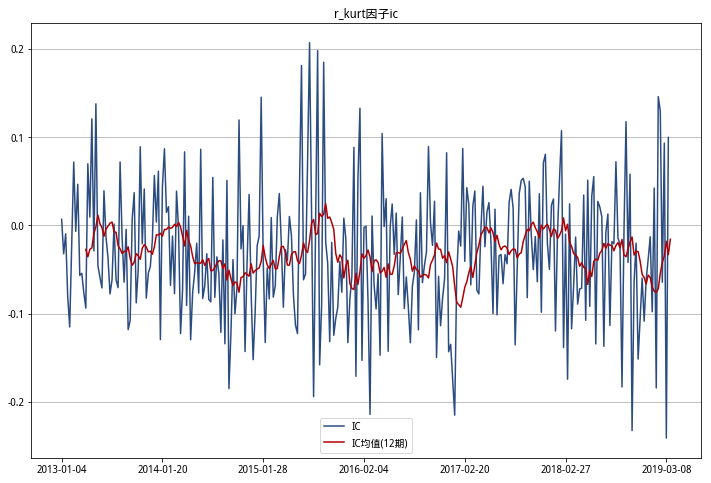## 4因子换手率

```# 展示某因子的换手率
def show_factor_turnover_rate(factor_dict, factor_name):
y_list = [[], []]

# 收益率折线图从0开始展开代码 ↓    ```
```# 'r_vol', 'r_skew', 'r_kurt'
show_factor_turnover_rate(factor_dict, 'r_vol')
show_factor_turnover_rate(factor_dict, 'r_skew')
show_factor_turnover_rate(factor_dict, 'r_kurt')
```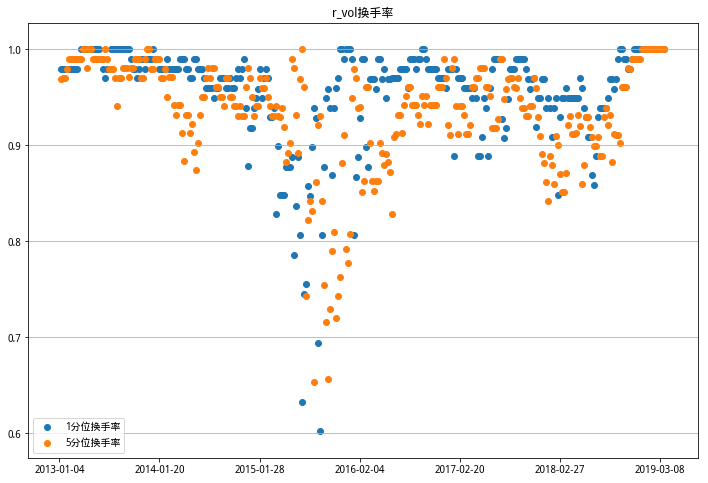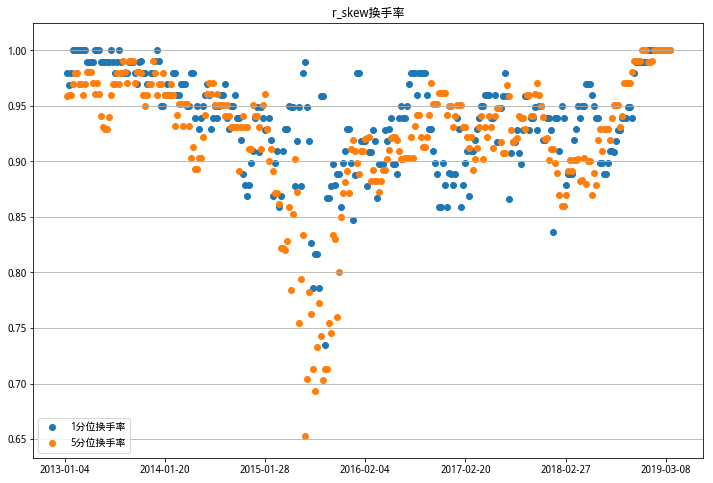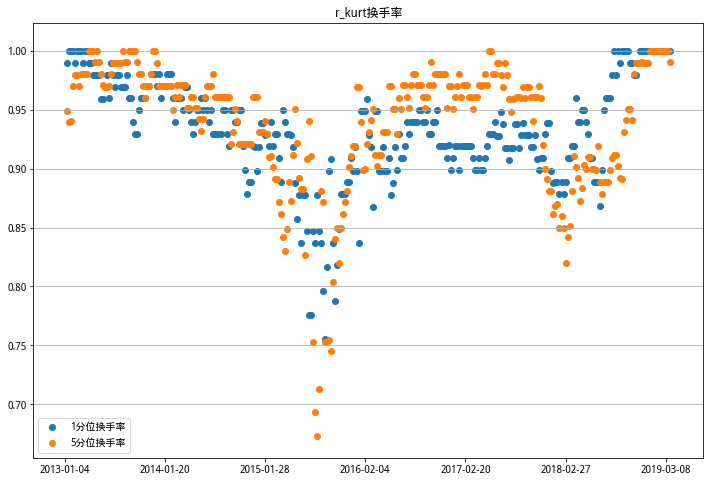# 6总结#### 全部评论